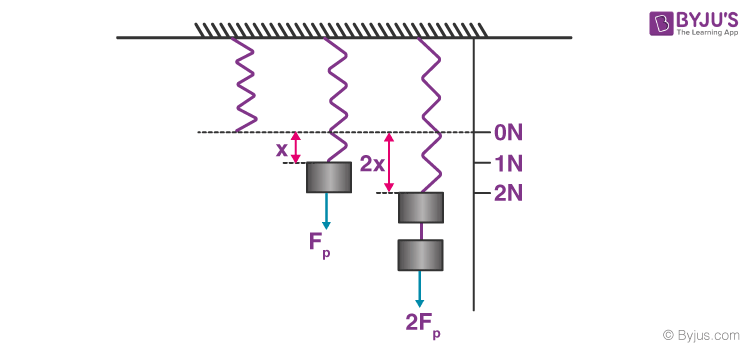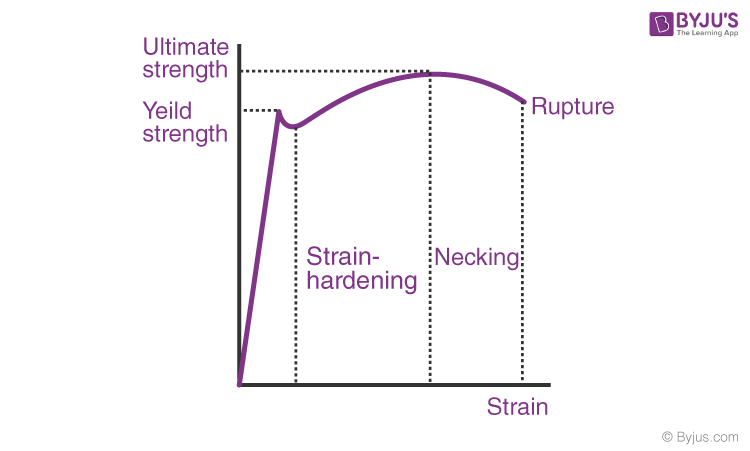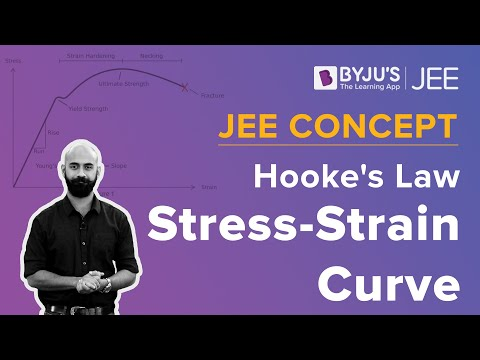# Hooke's Law

In the 19th-century, while studying springs and elasticity, English scientist Robert Hooke noticed that many materials exhibited a similar property when the stress-strain relationship was studied. There was a linear region where the force required to stretch the material was proportional to the extension of the material. This is known as Hooke’s Law. In this article, let us learn about Hooke’s law in detail.

## What is Hooke’s Law?

Stress and strain take different forms in different situations.Generally, for small deformations, the stress and strain are proportional to each other, and this is known as Hooke’s Law.

Hooke’s law states that the strain of the material is proportional to the applied stress within the elastic limit of that material.

When the elastic materials are stretched, the atoms and molecules deform until stress is applied, and when the stress is removed, they return to their initial state.

Mathematically, Hooke’s law is expressed as:

F = –kx

In the equation,F is the force, x is the extension length, k is the constant of proportionality known as spring constant in N/m.

### Hooke’s Law Experiment

Consider a spring with load application, as shown in the figure.Depending on the material, different springs will have different spring constants, which can be calculated. The figure shows us three instances, the stable condition of the spring, the spring elongated to an amount x under a load of 1 N, and the spring elongated to 2x under a load of 2 N. If we substitute these values in the Hooke’s law equation, we get the spring constant for the material in consideration.

### Hooke’s Law Solved Example

A spring is displaced by 5 cm and held in place with a force of 500 N. What is the spring constant of the spring?

Solution:

We know that the spring is displaced by 5 cm, but the unit of the spring constant is Newtons per meter. This means that we have to convert the distance to meters.

Converting the distance to meters, we get

5 cm = 0.05 m

Now substituting the values in the equation, we get

F = –k.x

Now, we need to rework the equation so that we are calculating for the missing metric which is the spring constant, or k. Looking only at the magnitudes and therefore omitting the negative sign, we get

500 N/0.05 m = k

k = 10000 N/m

Therefore, the spring constant of the spring is 10000 N/m.

### Hooke’s Law Graph

The figure below shows the stress-strain curve for low carbon steel.From the origin till the proportional limit nearing yield strength, the straight line implies that the material follows Hooke’s law. Beyond the elastic limit between proportional limit and yield strength, the material loses its elasticity and exhibits plasticity. The area under the curve from origin to the proportional limit falls under the elastic range. The area under the curve from a proportional limit to the rupture/fracture point falls under the plastic range.

The material’s ultimate strength is defined based on the maximum ordinate value given by the stress-strain curve (from origin to rupture). The value provides the rupture with strength at a point of rupture.

The video explains to you the general stress-strain graph of an elastic material experiencing tensile load and what are various stages in it. Within the elastic limit it follows Hooke’s law. As we keep on increasing the load, other stages and points like proportionality limit, yield point, fracture point, ultimate tensile strength are achieved, and they have been well explained in this video.## Hooke’s Law Applications

Following are some of the applications of Hooke’s Law:

• It is used as a fundamental principle behind the manometer, spring scale, the balance wheel of the clock.
• Hooke’s law sets the foundation for seismology, acoustics and molecular mechanics.

Following are some of the disadvantages of Hooke’s Law:

• Hooke’s law ceases to apply past the elastic limit of a material.
• Hooke’s law is accurate only for solid bodies if the forces and deformations are small.
• Hooke’s law isn’t a universal principle and only applies to the materials as long as they aren’t stretched way past their capacity.

## Frequently Asked Questions – FAQs

### Does Hooke’s Law apply to all materials?

Hooke’s spring law applies to any elastic object of arbitrary complexity, as long as a single number can express the deformation and the stress.

### Is Hooke’s Law linear?

Hooke’s Law is linear. Hooke’s law states that the restoring force is proportional to the displacement.

### When does Hooke’s Law fail?

Hooke’s law applies to a perfectly elastic material and does not apply beyond the elastic limit of any material.

### When is Hooke’s Law negative?

In Hooke’s law, the negative sign on the spring’s force means that the force exerted by the spring opposes the spring’s displacement.

### Why do we need Hooke’s Law?

Hooke’s Law is essential because it helps us understand how a stretchy object will behave when stretched or compacted.

Stay tuned to BYJU’S and Fall in Love with Learning!

Test Your Knowledge On Hookes Law Equation Experiment!